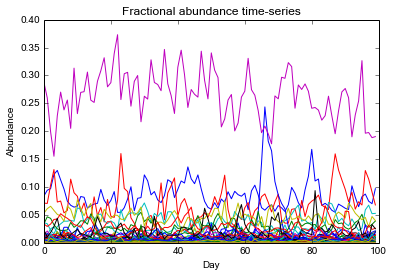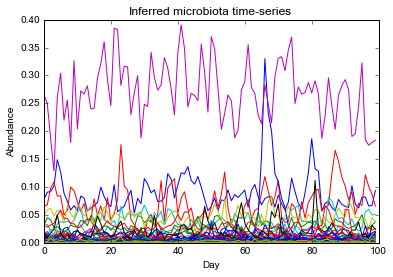# Normalizing microbiota time-series data¶

A demonstration of our normalization approach. This technique aims to reduce the number of spurious correlations inferred between bacterial taxa and host metadata by generating relative estimates of total bacterial load between sampling time points (see manuscript methods for more details). We will illustrate the procedure in 3 steps: 1) simulating a microbiota time-series and unequal sequencing of it; 2) applying our normalization process; 3) comparing our results to the true community abundances.

## 1. Simulate microbiota time-series¶

### Simulate the 'true' time-series, whose values correspond to actual abundances of bacterial taxa¶

Import Ornstein-Uhlenbeck (OU) process parameters inferred from Subject B's time-series data.

In :
import ExampleHelper # contains methods to facilitate this example
import pylab as pl

In :
ou_param_fn = './sim.ou_params.txt'
ou_param_M = np.genfromtxt(ou_param_fn)


Simulate OU process for each OTUs. Note that we simulate in log-space, since our Augmented Dickey Fuller testing suggests that most OTUs are stable in log-space.

In :
np.random.seed(1)
otu_d = {} # will store ou process for each simulated OTU
otu_c = ou_param_M.shape
for otu_i in range(otu_c):

# OU parameters for a given process
mu_n = ou_param_M[otu_i,0]
lambda_n = ou_param_M[otu_i,1]
sigma_n = ou_param_M[otu_i,2]
dt_n = ou_param_M[otu_i,3]
ou_v, t_v = ExampleHelper.OUProcess(x0=mu_n,mu_n=mu_n,sigma_n=sigma_n,lambda_n=lambda_n,dt_n=dt_n)

# join processes
otu_d[otu_i] = ou_v

#endfor


Convert log-space values to ones in linear space. The resulting time-series will be considered the 'true' bacterial abundances.

In :
time_c = len(otu_d)
abun_M = np.zeros((time_c,otu_c))
for i in otu_d:
abun_M[:,i] = np.power(10,otu_d[i])
#endfor


Plot the simulated microbiota time-series

In :
# Linear scale
pl.figure()
pl.plot(abun_M)
pl.xlabel('Day')
pl.ylabel('Abundance')
pl.title('Simulated microbiota time-series')

Out:
<matplotlib.text.Text at 0x112427e90>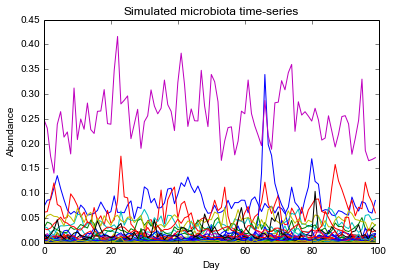In :
# Log-scale
pl.figure()
for otu_i in otu_d.keys():
pl.plot(otu_d[otu_i])
#endfor
pl.xlabel('Day')
pl.ylabel('log10(Abundance)')
pl.title('Simulated microbiota time-series')

Out:
<matplotlib.text.Text at 0x1138bc3d0>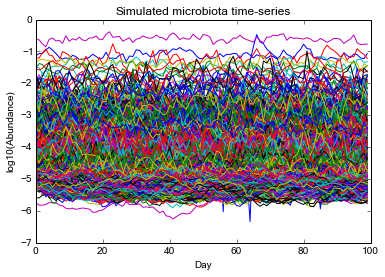### Simulate reads coming from sequencing of microbiota time-series¶

Simulate counts by drawing randomly from probability distribution defined by relative OTU abundances at each time point.

In :
read_count_fn = './sim.read_counts.txt'

In :
# obtain matrix of fractional abundances
sum_v = np.sum(abun_M,1)
sum_M = np.tile(sum_v,(len(otu_d),1)).T
frac_M = abun_M/sum_M

In :
# draw uniformly from cumulative probability distribution to randomly generate reads
seq_M = np.zeros(frac_M.shape) # will simulate sequencing reads
cumsum_M = np.cumsum(frac_M,1)

# step through each time point
for time_i in range(time_c):

# randomly pick a number of reads to use

# draw as many random numbers as chosen number of reads

# for each random number/read, pick OTU using cumulative probability
for rand_n in rand_v:
rand_i = np.min(np.nonzero(cumsum_M[time_i,:] > rand_n))
seq_M[time_i,rand_i] += 1.
#endfor
print "Simulating time point " + str(time_i)

#endfor

Simulating time point 0
Simulating time point 1
Simulating time point 2
Simulating time point 3
Simulating time point 4
Simulating time point 5
Simulating time point 6
Simulating time point 7
Simulating time point 8
Simulating time point 9
Simulating time point 10
Simulating time point 11
Simulating time point 12
Simulating time point 13
Simulating time point 14
Simulating time point 15
Simulating time point 16
Simulating time point 17
Simulating time point 18
Simulating time point 19
Simulating time point 20
Simulating time point 21
Simulating time point 22
Simulating time point 23
Simulating time point 24
Simulating time point 25
Simulating time point 26
Simulating time point 27
Simulating time point 28
Simulating time point 29
Simulating time point 30
Simulating time point 31
Simulating time point 32
Simulating time point 33
Simulating time point 34
Simulating time point 35
Simulating time point 36
Simulating time point 37
Simulating time point 38
Simulating time point 39
Simulating time point 40
Simulating time point 41
Simulating time point 42
Simulating time point 43
Simulating time point 44
Simulating time point 45
Simulating time point 46
Simulating time point 47
Simulating time point 48
Simulating time point 49
Simulating time point 50
Simulating time point 51
Simulating time point 52
Simulating time point 53
Simulating time point 54
Simulating time point 55
Simulating time point 56
Simulating time point 57
Simulating time point 58
Simulating time point 59
Simulating time point 60
Simulating time point 61
Simulating time point 62
Simulating time point 63
Simulating time point 64
Simulating time point 65
Simulating time point 66
Simulating time point 67
Simulating time point 68
Simulating time point 69
Simulating time point 70
Simulating time point 71
Simulating time point 72
Simulating time point 73
Simulating time point 74
Simulating time point 75
Simulating time point 76
Simulating time point 77
Simulating time point 78
Simulating time point 79
Simulating time point 80
Simulating time point 81
Simulating time point 82
Simulating time point 83
Simulating time point 84
Simulating time point 85
Simulating time point 86
Simulating time point 87
Simulating time point 88
Simulating time point 89
Simulating time point 90
Simulating time point 91
Simulating time point 92
Simulating time point 93
Simulating time point 94
Simulating time point 95
Simulating time point 96
Simulating time point 97
Simulating time point 98
Simulating time point 99


## 2. Normalize simulated sequencing data¶

Here, we normalize the simulated sequence data. We will first estimate the expected abundance of each OTU at each time point. These abundances are estimated from other samples, which are weighted according to their similarity to the time point of interest. (We use the Jensen-Shannon Distance to compute a weighting). Next, we regress OTU abundances observed at each time point against their expected abundances; the slope (computed using a median, so as to be robust to outliers) is ultimately used as a scaling factor to estimate relative overall bacterial loads.

### Compute sample pairwise similarity matrices using Jensen-Shannon Distance¶

In :
import scipy.spatial.distance as ssd
seq_sum_v = np.sum(seq_M,1)
seq_frac_M = seq_M/np.tile(seq_sum_v,(otu_c,1)).T
jsd_M = ssd.squareform(ssd.pdist(seq_frac_M,ExampleHelper.JSD))
dist_M = np.sqrt(jsd_M)


### Perform normalization¶

Setup weighting matrix using JSD. Use simple scheme based on squares.

In :
weight_M = np.zeros((time_c,time_c)) + np.nan
time_v = range(time_c)
for time_i in time_v:
weight_v = np.power(1 - jsd_M[time_i,:],2)
weight_v = weight_v/np.sum(weight_v)
weight_M[time_i,:] = weight_v
weight_M[time_i,time_i] = 0.
#endfor


Simplify by looking at most abundant OTUs (defined as the fewest number tha account for 90% of reads)

In :
median_v = np.median(seq_M,0)
frac_v = median_v/sum(median_v)
sort_v = np.flipud(np.sort(frac_v))
cumsum_v = np.cumsum(sort_v)
thresh_i = np.nonzero(cumsum_v > 0.9)
otu_subset_v = np.nonzero(frac_v > sort_v[thresh_i])


Start the normalization using fractional abundances converted to log-space

In :
obs_M = np.log10(seq_frac_M[:,otu_subset_v])
start_M = obs_M.copy()


Normalize each time point, in random order

In :
random.shuffle(time_v)
for t in time_v:

# observed abundances
obs_v = obs_M[t,:]

# expected abundances
exp_v = ExampleHelper.WeightedMedian(obs_M,weight_M,t)

# solve y=mx in log-space, using median, so as to avoid effects of outliers
m_t = np.median(obs_v-exp_v)

# update time-series
obs_M[t,:] = obs_M[t,:] - m_t

#endfor


Now, rescale simulated sequence data using inferred scaling factors.

In :
scale_v = start_M[:,0]-obs_M[:,0] # scaling factors for each time point
scale_M = np.tile(scale_v,(seq_frac_M.shape,1)).T
scale_frac_M = np.log10(seq_frac_M) - scale_M
inf_abun_M = np.power(10,scale_frac_M) # inferred OTU abundances in linear space


## 3. Compare inferred results to true answer¶

Note that the inferred abundances are not expected to estime the TRUE abundance of bacteria at each time point, but rather the RELATIVE abundance of bacteria BETWEEN time points. Therefore, to make the clearest comparison of the true and estimated total bacterial abundances, we have scaled the time-series of inferred abundances to have the same mean as the time-series of true abundances.

In :
# estimate total community abundances at each time point.
true_abun_v = np.sum(abun_M,1)
inf_abun_v = np.sum(inf_abun_M,1)

# scale inferred abundances so that shares the same mean with the true abundances.
# this will not affect RELATIVE abundances between each time point
inf_abun_v += (np.mean(true_abun_v)-np.mean(inf_abun_v))


Show both a time-series and scatter plot of TRUE and INFERRED bacterial abundances. Note that we will not compute a standard correlation here, as the resulting p-values will be artificially low. (See notes in Methods on spurious cross-correlations due to auto-correlated data; in this case, the community abundances are auto-correlated.)

In :
pl.plot(inf_abun_v,'-ob',label='Inferred')
pl.plot(true_abun_v,'-or',label='True')
pl.xlabel('Day')
pl.ylabel('Total bacterial abundances')
pl.title('True and inferred bacterial abundances over time')
pl.grid()
pl.legend()

Out:
<matplotlib.legend.Legend at 0x1151c80d0>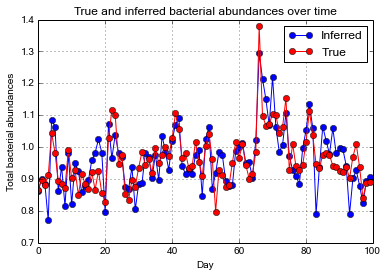Scatter plot of inferred and true total bacterial abundances on each day

In :
pl.figure(figsize=(4,4))
pl.plot(inf_abun_v,true_abun_v,'o')
pl.grid()
pl.xlim([0.7,1.3])
pl.ylim([0.7,1.3])
pl.xlabel('Inferred')
pl.ylabel(True)
pl.title('Scatter plot of True and Inferred total bacterial abundances')

Out:
<matplotlib.text.Text at 0x11529fb10>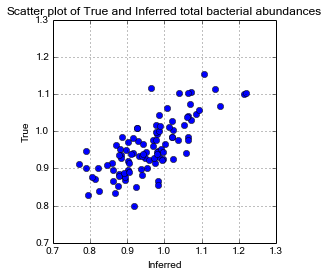We show below the simulated, fractional, and normalized (via our method) OTU abundances as a final demonstration of our method's performance. Note how near Day 65, in the true dataset, there is a spike in the abundance of the blue OTU (upper plot). Conventional normalization techniques (i.e. converting to fractional abundances) suggest the Day 65 spike in the blue OTU is accompanied by a decrease in the abundance of the purple OTU (middle plot). However, our technique correctly infers solely a Day 65 increase in the blue OTU, and not a large decrease in the abundance of the purple OTU (bottom plot).

In :
# Simulated
pl.figure()
pl.plot(abun_M)
pl.xlabel('Day')
pl.ylabel('Abundance')
pl.title('Simulated microbiota time-series')

# Fractional abundances
pl.figure()
pl.plot(seq_frac_M);
pl.title('Fractional abundance time-series')
pl.xlabel('Day')
pl.ylabel('Abundance')

# Our normalized abundances
pl.figure()
pl.plot(inf_abun_M);
pl.title('Inferred microbiota time-series')
pl.xlabel('Day')
pl.ylabel('Abundance')

Out:
<matplotlib.text.Text at 0x119540390>